Open In App

# Josephus Problem

There are N people standing in a circle waiting to be executed. The counting out begins at some point in the circle and proceeds around the circle in a fixed direction. In each step, a certain number of people are skipped and the next person is executed. The elimination proceeds around the circle (which is becoming smaller and smaller as the executed people are removed), until only the last person remains, who is given freedom.

Given the total number of persons N and a number k which indicates that k-1 persons are skipped and the kth person is killed in a circle. The task is to choose the person in the initial circle that survives.

Examples:

Input: N = 5 and k = 2
Output: 3
Explanation: Firstly, the person at position 2 is killed,
then the person at position 4 is killed, then the person at position 1 is killed.
Finally, the person at position 5 is killed. So the person at position 3 survives.

Input: N = 7 and k = 3
Output: 4
Explanations: The persons at positions 3, 6, 2, 7, 5, and 1 are killed in order,
and the person at position 4 survives.

## Josephus problem using List:

The simple approach is to create a list and add all values from 1 to N to it. Create a recursive function that takes a list, start (position at which counting will start), and k ( number of people to be skipped) as an argument. If the size of the list is one i.e. only one person left then return this position. Otherwise, start counting the k person in a clockwise direction from starting position and remove the person at the kth position. Now the person at the kth position is removed and now counting will start from this position. This process continues till only one person is left.

### Pseudocode :

Josephus( list , start , k){
if list.size = 1
return list
start = (start + k) % list.size
list.remove( start )
return Josephus( list, start, k)
}

Follow the below steps to Implement the idea:

• Create a vector person and push all the values from 1 to N in person.
• Recursively eliminate the index element
• Erase the element on the position index.
• Call for (index + k)% size of person.
• If size of person = 1, return person[i].

Below is the Implementation of the above approach:

## C++

 `#include ` `using` `namespace` `std;` `void` `Josh(vector<``int``> person, ``int` `k, ``int` `index)``{``    ``// Base case , when only one person is left``    ``if` `(person.size() == 1) {``        ``cout << person << endl;``        ``return``;``    ``}` `    ``// find the index of first person which will die``    ``index = ((index + k) % person.size());` `    ``// remove the first person which is going to be killed``    ``person.erase(person.begin() + index);` `    ``// recursive call for n-1 persons``    ``Josh(person, k, index);``}` `int` `main()``{``    ``int` `n = 14; ``// specific n and k  values for original``                ``// josephus problem``    ``int` `k = 2;``    ``k--; ``// (k-1)th person will be killed``    ``int` `index``        ``= 0; ``// The index where the person which will die` `    ``vector<``int``> person;``    ``// fill the person vector``    ``for` `(``int` `i = 1; i <= n; i++) {``        ``person.push_back(i);``    ``}` `    ``Josh(person, k, index);``}`

## Java

 `import` `java.util.*;` `class` `GFG{`  `  ``static` `void` `Josh(List person, ``int` `k, ``int` `index)``  ``{``    ` `    ``// Base case , when only one person is left``    ``if` `(person.size() == ``1``) {``      ``System.out.println(person.get(``0``));``      ``return``;``    ``}` `    ``// find the index of first person which will die``    ``index = ((index + k) % person.size());` `    ``// remove the first person which is going to be killed``    ``person.remove(index);` `    ``// recursive call for n-1 persons``    ``Josh(person, k, index);``  ``}` `  ``// Driver code``  ``public` `static` `void` `main(String [] args)``  ``{``    ``int` `n = ``14``; ``// specific n and k  values for original``    ``// josephus problem``    ``int` `k = ``2``;``    ``k--; ``// (k-1)th person will be killed``    ``int` `index``      ``= ``0``; ``// The index where the person which will die` `    ``List person = ``new` `ArrayList<>();``    ` `    ``// fill the person vector``    ``for` `(``int` `i = ``1``; i <= n; i++) {``      ``person.add(i);``    ``}` `    ``Josh(person, k, index);``  ``}``}` `// This code is contributed by umadevi9616`

## Python3

 `# Python code for Josephus Problem``def` `Josh(person, k, index):``  ` `  ``# Base case , when only one person is left``  ``if` `len``(person) ``=``=` `1``:``    ``print``(person[``0``])``    ``return``  ` `  ``# find the index of first person which will die``  ``index ``=` `((index``+``k)``%``len``(person))``  ` `   ``# remove the first person which is going to be killed``  ``person.pop(index)``  ` `  ``# recursive call for n-1 persons``  ``Josh(person,k,index)` `# Driver Program to test above function``n ``=` `14` `# specific n and k  values for original josephus problem``k ``=` `2``k``-``=``1`   `# (k-1)th person will be killed` `index ``=` `0` `# fill the person vector``person``=``[]``for` `i ``in` `range``(``1``,n``+``1``):``  ``person.append(i)` `Josh(person,k,index)` `# This code is contributed by``# Gaurav Kandel`

## C#

 `using` `System;``using` `System.Collections.Generic;``class` `GFG {``    ` `    ``static` `void` `Josh(List<``int``> person, ``int` `k, ``int` `index)``    ``{``        ``// Base case , when only one person is left``        ``if` `(person.Count == 1) {``            ``Console.WriteLine(person);``            ``return``;``        ``}``     ` `        ``// find the index of first person which will die``        ``index = ((index + k) % person.Count);``     ` `        ``// remove the first person which is going to be killed``        ``person.RemoveAt(index);``     ` `        ``// recursive call for n-1 persons``        ``Josh(person, k, index);``    ``}` `  ``// Driver code``  ``static` `void` `Main()``  ``{``    ``int` `n = 14; ``// specific n and k  values for original``                ``// josephus problem``    ``int` `k = 2;``    ``k--; ``// (k-1)th person will be killed``    ``int` `index``        ``= 0; ``// The index where the person which will die`` ` `    ``List<``int``> person = ``new` `List<``int``>();``    ``// fill the person vector``    ``for` `(``int` `i = 1; i <= n; i++) {``        ``person.Add(i);``    ``}`` ` `    ``Josh(person, k, index);``  ``}``}` `// This code is contributed by divyesh072019.`

## Javascript

 ``

Output

`13`

Time Complexity: O(N2)
Auxiliary Space: O(N), For recursion stack

## Approach to solve Josephus problem iteratively:

Illustration:

N = 5,  k = 2

Add all values from 1 to N in the list. We will call the recursive function with start = 0 and k = 1 (0-indexing)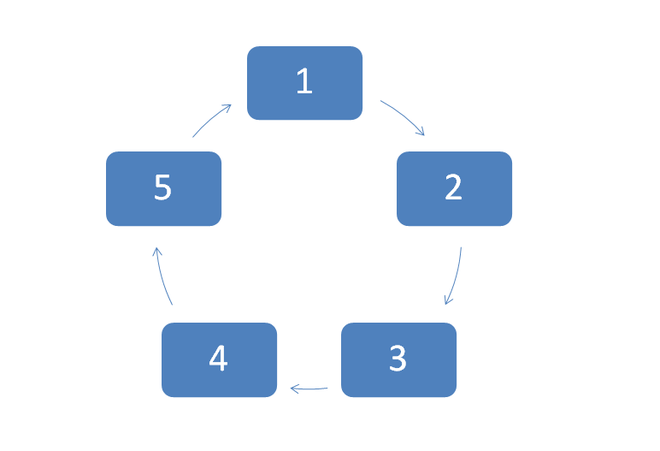Now the element at 1-index (person number 2) will be killed. And it is removed from the list. The new counting will begin from 1-index, the person at 1-index killed so now person at 2-index (person number 3) comes to 1-index and counting starts from here now.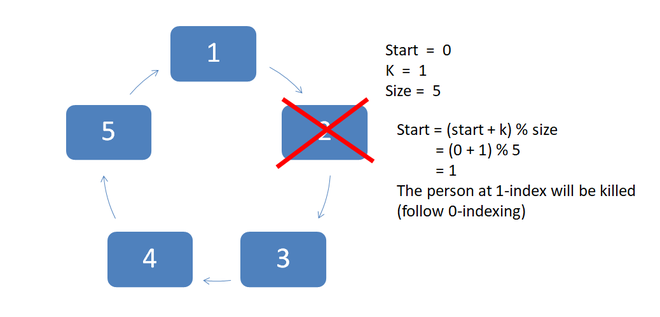Now we have 4 people, counting starting from 1-index (person number 3) and the person at kth (2-index ) position will be killed.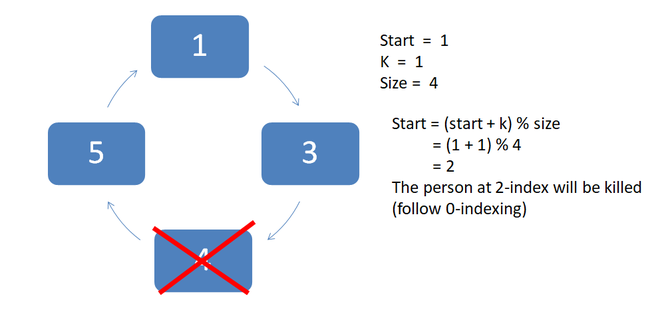The person at 2-index (person number 4) was killed so now we have 3 people left and the person (person number 5) at 3-index shifted to 2-index. And counting starts from here.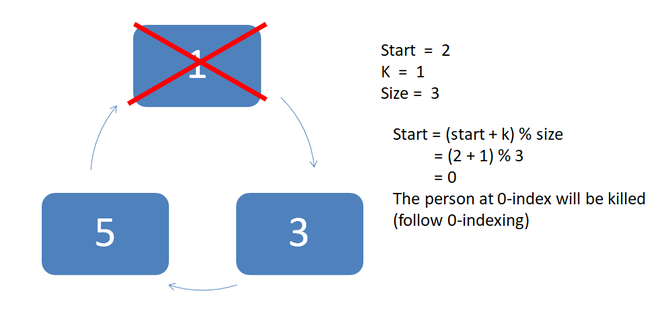The person at the 0-index was killed and we have now two-person left in the circle. And the person at 1-index shifted to 0-index i.e. person number 3.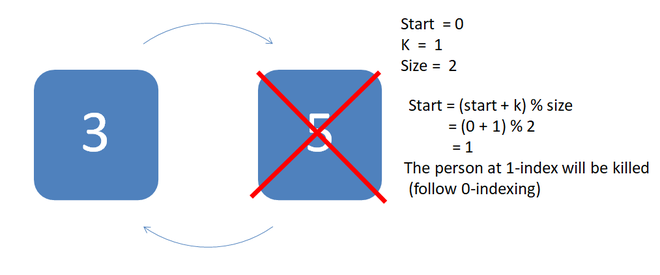Final counting done and the person at 1-index killed and the only person who is left is at position 3.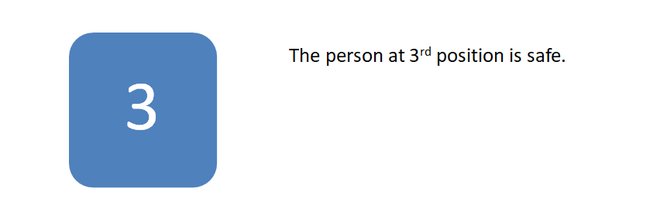Follow the below steps to Implement the idea:

• Initialize variables num, cnt, and cut with 1, 0, and 0 respectively and an array arr[] of size N with the initial value set as 1.
• Run a while loop till cnt < N:
• Run a while loop till num is less than equal to k.
• Increment cut by one and take modulo by N
• If arr[cut] = 1 increment num by one.
•  Set num = 1, arr[cut] = 0 and increment cnt and cut by one and cut = cut % n;
• Run a while loop till arr[cut] = 0 and increment cut by one.
• Return cnt + 1 as the required answer.

Below is the Implementation of the above approach:

## C++

 `#include ``using` `namespace` `std;` `int` `Josephus(``int``, ``int``);` `int` `Josephus(``int` `n, ``int` `k)``{``    ``k--;``    ``int` `arr[n];` `    ``// Makes all the 'n' people alive by``    ``// assigning them value = 1``    ``for` `(``int` `i = 0; i < n; i++) {``        ``arr[i] = 1;``    ``}``    ``int` `cnt = 0, cut = 0,``        ``// Cut = 0 gives the sword to 1st person.``        ``num = 1;` `    ``// Loop continues till n-1 person dies.``    ``while` `(cnt < (n - 1)) {` `        ``// Checks next (kth) alive persons.``        ``while` `(num <= k) {``            ``cut++;` `            ``// Checks and resolves overflow``            ``// of Index.``            ``cut = cut % n;``            ``if` `(arr[cut] == 1) {``                ``// Updates the number of persons``                ``// alive.``                ``num++;``            ``}``        ``}` `        ``// Refreshes value to 1 for next use.``        ``num = 1;` `        ``// Kills the person at position of 'cut'``        ``arr[cut] = 0;` `        ``// Updates the no. of killed persons.``        ``cnt++;``        ``cut++;` `        ``// Checks and resolves overflow of Index.``        ``cut = cut % n;` `        ``// Checks the next alive person the``        ``// sword is to be given.``        ``while` `(arr[cut] == 0) {``            ``cut++;` `            ``// Checks and resolves overflow``            ``// of Index.``            ``cut = cut % n;``        ``}``    ``}` `    ``// Output is the position of the last``    ``// man alive(Index + 1);``    ``return` `cut + 1;``}` `// Driver code``int` `main()``{``    ``int` `n = 14, k = 2;``    ``cout << Josephus(n, k);``    ``return` `0;``}` `// THIS CODE IS PRESENTED BY SHISHANK RAWAT`

## Java

 `// Java code to implement the above approach``import` `java.io.*;``class` `GFG {` `    ``public` `static` `void` `main(String[] args)``    ``{``        ``int` `n = ``14``, k = ``2``;``        ``System.out.println(Josephus(n, k));``    ``}` `    ``public` `static` `int` `Josephus(``int` `n, ``int` `k)``    ``{``        ``k--;``        ``int` `arr[] = ``new` `int``[n];``        ``for` `(``int` `i = ``0``; i < n; i++) {``            ``arr[i] = ``1``; ``// Makes all the 'n' people alive by``            ``// assigning them value = 1``        ``}``        ``int` `cnt = ``0``, cut = ``0``,``            ``num``            ``= ``1``; ``// Cut = 0 gives the sword to 1st person.``        ``while` `(``            ``cnt``            ``< (n``               ``- ``1``)) ``// Loop continues till n-1 person dies.``        ``{``            ``while` `(num``                   ``<= k) ``// Checks next (kth) alive persons.``            ``{``                ``cut++;``                ``cut = cut``                      ``% n; ``// Checks and resolves overflow``                ``// of Index.``                ``if` `(arr[cut] == ``1``) {``                    ``num++; ``// Updates the number of persons``                    ``// alive.``                ``}``            ``}``            ``num = ``1``; ``// refreshes value to 1 for next use.``            ``arr[cut] = ``0``; ``// Kills the person at position of``                          ``// 'cut'``            ``cnt++; ``// Updates the no. of killed persons.``            ``cut++;``            ``cut = cut % n; ``// Checks and resolves overflow``                           ``// of Index.``            ``while` `(arr[cut]``                   ``== ``0``) ``// Checks the next alive person the``            ``// sword is to be given.``            ``{``                ``cut++;``                ``cut = cut``                      ``% n; ``// Checks and resolves overflow``                ``// of Index.``            ``}``        ``}``        ``return` `cut``            ``+ ``1``; ``// Output is the position of the last``        ``// man alive(Index + 1);``    ``}``}` `// This code is contributed by Shubham Singh`

## Python3

 `def` `Josephus(n, k):``    ` `    ``k ``-``=` `1``    ``arr ``=` `[``0``]``*``n``    ``for` `i ``in` `range``(n):``        ``arr[i] ``=` `1` `# Makes all the 'n' people alive by``        ``# assigning them value = 1``    ``cnt ``=` `0``    ``cut ``=` `0``    ``num ``=` `1` `# Cut = 0 gives the sword to 1st person.``    ``while` `(cnt < (n ``-` `1``)):``      ` `        ``# Loop continues till n-1 person dies.``        ``while` `(num <``=` `k):``          ` `            ``# Checks next (kth) alive persons.``            ``cut ``+``=` `1``            ``cut ``=` `cut ``%` `n ``# Checks and resolves overflow``            ``# of Index.``            ``if` `(arr[cut] ``=``=` `1``):``                ``num``+``=``1` `# Updates the number of persons``                ``# alive.``        ` `        ``num ``=` `1` `# refreshes value to 1 for next use.``        ``arr[cut] ``=` `0` `# Kills the person at position of 'cut'``        ``cnt ``+``=` `1` `# Updates the no. of killed persons.``        ``cut ``+``=` `1``        ``cut ``=` `cut ``%` `n ``# Checks and resolves overflow of Index.``        ``while` `(arr[cut] ``=``=` `0``):``          ` `            ``# Checks the next alive person the``            ``# sword is to be given.``            ``cut ``+``=` `1``            ``cut ``=` `cut ``%` `n ``# Checks and resolves overflow``            ``# of Index.``    ` `    ``return` `cut ``+` `1` `# Output is the position of the last``                    ``# man alive(Index + 1)` `# Driver Code``n, k ``=` `14``, ``2` `#map (int, input().splut())``print``(Josephus(n, k))` `# This code is contributed by ShubhamSingh`

## C#

 `// C# code to implement the above approach``using` `System;``using` `System.Linq;` `public` `class` `GFG{` `  ``public` `static` `void` `Main ()``  ``{``    ``int` `n = 14, k = 2;``    ``Console.Write(Josephus(n, k));``  ``}` `  ``public` `static` `int` `Josephus(``int` `n, ``int` `k)``  ``{``    ``k--;``    ``int``[] arr = ``new` `int``[n];``    ``for` `(``int` `i = 0; i < n; i++) {``      ``arr[i] = 1; ``// Makes all the 'n' people alive by``      ``// assigning them value = 1``    ``}``    ``int` `cnt = 0, cut = 0,``    ``num = 1; ``// Cut = 0 gives the sword to 1st person.``    ``while` `(``      ``cnt``      ``< (n - 1)) ``// Loop continues till n-1 person dies.``    ``{``      ``while` `(num <= k) ``// Checks next (kth) alive persons.``      ``{``        ``cut++;``        ``cut = cut % n;``        ` `        ``// Checks and resolves overflow``        ``// of Index.``        ``if` `(arr[cut] == 1)``        ``{``          ``num++; ``// Updates the number of persons``          ``// alive.``        ``}``      ``}``      ``num = 1; ``// refreshes value to 1 for next use.``      ``arr[cut]``        ``= 0; ``// Kills the person at position of 'cut'``      ``cnt++; ``// Updates the no. of killed persons.``      ``cut++;``      ``cut = cut``        ``% n; ``// Checks and resolves overflow of Index.``      ``while` `(arr[cut]``             ``== 0) ``// Checks the next alive person the``        ``// sword is to be given.``      ``{``        ``cut++;``        ``cut = cut % n; ``// Checks and resolves overflow``        ``// of Index.``      ``}``    ``}``    ``return` `cut + 1; ``// Output is the position of the last``    ``// man alive(Index + 1);``  ``}``}` `// This code is contributed by Shubham Singh`

## Javascript

 ``

Output

`13`

Time Complexity: O(N2)
Auxiliary Space: O(N)

## Josephus Problem in Linear Time and Constant Space:

Follow the below steps to Solve the Problem (Approach):

• Initialize variables i and ans with 1 and 0 respectively.
• Run a while loop till i <= N:
• Update ans with (ans + k) % i.
• Increment i by 1.
• Return ans + 1 as the required answer.

Below is the Implementation of the above Steps:

## C++

 `// C++ code to Implement Josephus Problem` `#include ``using` `namespace` `std;` `int` `Josephus(``int` `N, ``int` `k)``{` `    ``// Initialize variables i and ans with 1 and 0``    ``// respectively.` `    ``int` `i = 1, ans = 0;` `    ``// Run a while loop till i <= N` `    ``while` `(i <= N) {` `        ``// Update the Value of ans and Increment i by 1``        ``ans = (ans + k) % i;``        ``i++;``    ``}` `    ``// Return required answer``    ``return` `ans + 1;``}` `// main function``int` `main()``{` `    ``int` `N = 14, k = 2;``    ``cout << Josephus(N, k) << endl;``    ``return` `0;``}` `// This code is presented by Akash Mangal`

## C

 `// C Program to Implement Josephus Problem` `#include ` `int` `Josephus(``int` `N, ``int` `k)``{` `    ``// Initialize variables i and ans with 1 and 0``    ``// respectively.` `    ``int` `i = 1, ans = 0;` `    ``// Run a while loop till i <= N` `    ``while` `(i <= N) {` `        ``// Update the Value of ans and Increment i by 1``        ``ans = (ans + k) % i;``        ``i++;``    ``}` `    ``// Return required answer``    ``return` `ans + 1;``}` `// main function``int` `main()``{` `    ``int` `N = 14, k = 2;``    ``printf``(``"%d"``, Josephus(N, k));``    ``return` `0;``}` `// This code is presented by Akash Mangal`

## Java

 `// Java code to Implement Josephus Problem``import` `java.io.*;` `class` `GFG {``  ``public` `static` `int` `Josephus(``int` `N, ``int` `k) {` `    ``// Initialize variables i and ans with 1 and 0 respectively.``    ``int` `i = ``1``, ans = ``0``;` `    ``// Run a while loop till i <= N``    ``while` `(i <= N) {` `      ``// Update the Value of ans and Increment i by 1``      ``ans = (ans + k) % i;``      ``i++;``    ``}` `    ``// Return required answer``    ``return` `ans + ``1``;``  ``}`  `  ``// main function``  ``public` `static` `void` `main (String[] args) {` `    ``int` `N = ``14``, k = ``2``;``    ``int` `ans = Josephus(N, k);``    ``System.out.println(ans);``  ``}``}` `// This code is presented by Akash Mangal`

## Python

 `# python code to implement Josephus problem` `# Josephus function which will take``# two parameter N and K, number of people and positions respectively``# return the position of person survives``def` `Josephus(n, k):` `    ``# initialize two variables i and ans``    ``i ``=` `1``    ``ans ``=` `0``    ``while``(i <``=` `n):` `        ``# update the value of ans``        ``ans ``=` `(ans ``+` `k) ``%` `i``        ``i ``+``=` `1``    ` `    ``# returning the required answer``    ``return` `ans ``+` `1` `# driver code``# let``n ``=` `14``k ``=` `2` `result ``=` `Josephus(n, k)``print``(result)` `# This code is contributed by sarveshc111.`

## Javascript

 `// driver code``let n = 14, k = 2;``document.write(Josephus(n,k));` `// Josephus function``// return the position of last man survives``function` `Josephus(n, k)``{``    ``let i = 1, ans = 0;``    ``while``(i <= n ){` `        ``// update the value of ans``        ``ans = (ans + k) % i;``        ``i++;``    ``}``    ``return` `ans + 1;``}` `// This code is contributed by sarveshc111.`

## C#

 `// C# code to Implement Josephus Problem``using` `System;` `class` `GFG``{``    ``public` `static` `int` `Josephus(``int` `N, ``int` `k)``    ``{``        ``// Initialize variables i and ans with 1 and 0 respectively.``        ``int` `i = 1, ans = 0;` `        ``// Run a while loop till i <= N``        ``while` `(i <= N)``        ``{``            ``// Update the Value of ans and Increment i by 1``            ``ans = (ans + k) % i;``            ``i++;``        ``}` `        ``// Return required answer``        ``return` `ans + 1;``    ``}`  `    ``// main function``    ``static` `void` `Main(``string``[] args)``    ``{``        ``int` `N = 14, k = 2;``        ``int` `ans = Josephus(N, k);``        ``Console.WriteLine(ans);``    ``}``}`

Output

`13`

Time Complexity: O(N)
Auxiliary Space: O(1)

## Josephus Problem using Recursion:

Below is the idea to solve the problem:

The problem has the following recursive structure. josephus(n, k) = (josephus(n – 1, k) + k-1) % n + 1 and josephus(1, k) = 1

After the first person (kth from the beginning) is killed, n-1 persons are left. Make recursive call for Josephus(n – 1, k) to get the position with n-1 persons. But the position returned by Josephus(n – 1, k) will consider the position starting from k%n + 1. So make adjustments to the position returned by Josephus(n – 1, k).

Below is the Implementation of the above idea.

## C++

 `// C++ code to implement the idea` `#include ``using` `namespace` `std;` `// Recursive function to implement the Josephus problem``int` `josephus(``int` `n, ``int` `k)``{``    ``if` `(n == 1)``        ``return` `1;``    ``else``        ``// The position returned by josephus(n - 1, k)``        ``// is adjusted because the recursive call``        ``// josephus(n - 1, k) considers the``        ``// original position k % n + 1 as position 1``        ``return` `(josephus(n - 1, k) + k - 1) % n + 1;``}` `// Driver code``int` `main()``{``    ``int` `n = 14;``    ``int` `k = 2;``    ``cout << ``"The chosen place is "` `<< josephus(n, k);``    ``return` `0;``}` `// This code is contributed by shubhamsingh10`

## C

 `#include ` `int` `josephus(``int` `n, ``int` `k)``{``    ``if` `(n == 1)``        ``return` `1;``    ``else``        ``/* The position returned by josephus(n - 1, k) is``           ``adjusted because the recursive call josephus(n -``           ``1, k) considers the original position``           ``k%n + 1 as position 1 */``        ``return` `(josephus(n - 1, k) + k - 1) % n + 1;``}` `// Driver Program to test above function``int` `main()``{``    ``int` `n = 14;``    ``int` `k = 2;``    ``printf``(``"The chosen place is %d"``, josephus(n, k));``    ``return` `0;``}`

## Java

 `// Java code for Josephus Problem``import` `java.io.*;` `class` `GFG {` `    ``static` `int` `josephus(``int` `n, ``int` `k)``    ``{``        ``if` `(n == ``1``)``            ``return` `1``;``        ``else``            ``/* The position returned by josephus(n - 1, k)``            ``is adjusted because the recursive call``            ``josephus(n - 1, k) considers the original``            ``position k%n + 1 as position 1 */``            ``return` `(josephus(n - ``1``, k) + k - ``1``) % n + ``1``;``    ``}` `    ``// Driver Program to test above function``    ``public` `static` `void` `main(String[] args)``    ``{``        ``int` `n = ``14``;``        ``int` `k = ``2``;``        ``System.out.println(``"The chosen place is "``                           ``+ josephus(n, k));``    ``}``}` `// This code is contributed by Prerna Saini`

## Python3

 `# Python code for Josephus Problem`  `def` `josephus(n, k):` `    ``if` `(n ``=``=` `1``):``        ``return` `1``    ``else``:` `        ``# The position returned by``        ``# josephus(n - 1, k) is adjusted``        ``# because the recursive call``        ``# josephus(n - 1, k) considers``        ``# the original position``        ``# k%n + 1 as position 1``        ``return` `(josephus(n ``-` `1``, k) ``+` `k``-``1``) ``%` `n ``+` `1` `# Driver Program to test above function`  `n ``=` `14``k ``=` `2` `print``(``"The chosen place is "``, josephus(n, k))` `# This code is contributed by``# Sumit Sadhakar`

## C#

 `// C# code for Josephus Problem``using` `System;` `class` `GFG {` `    ``static` `int` `josephus(``int` `n, ``int` `k)``    ``{``        ``if` `(n == 1)``            ``return` `1;``        ``else``            ``/* The position returned``            ``by josephus(n - 1, k) is``            ``adjusted because the``            ``recursive call josephus(n``            ``- 1, k) considers the``            ``original position k%n + 1``            ``as position 1 */``            ``return` `(josephus(n - 1, k) + k - 1) % n + 1;``    ``}` `    ``// Driver Program to test above``    ``// function``    ``public` `static` `void` `Main()``    ``{``        ``int` `n = 14;``        ``int` `k = 2;``        ``Console.WriteLine(``"The chosen "``                          ``+ ``"place is "` `+ josephus(n, k));``    ``}``}` `// This code is contributed by anuj_67.`

## PHP

 ``

## Javascript

 ``

Output

`The chosen place is 13`

Time Complexity: O(N)
Auxiliary Space: O(N) the space used in recursion call stack

Please visit set-2: Josephus problem | Set 2 (A Simple Solution when k = 2)

Source:
http://en.wikipedia.org/wiki/Josephus_problem

## Josephus Problem using  Queue :

### Approach:

I am using queue data structure, so that i can move the items from one end to another linearly.
we have given ‘K’ the moves we can shift, so after shifting K-1 items to the end of the queue, I am deleting the front element.
On repeating the above step, a stage will come when only one element will be left, this is answer itself.

## C++

 `#include ``#include // include the queue library``using` `namespace` `std;` `int` `findTheWinner(``int` `n, ``int` `k) {` `    ``queue<``int``> q; ``// create a queue to store the players` `    ``// add all players to the queue``    ``int` `i=1;``    ``while``(i<=n){``        ``q.push(i);``        ``i++;``    ``}` `    ``// loop until there is only one player left in the queue``    ``while``(q.size()!=1){` `        ``// move the first k-1 players from front to back of the``      ``//queue in circular manner``        ``int` `j=1;``        ``while``(j

## Java

 `import` `java.util.LinkedList;``import` `java.util.Queue;` `public` `class` `Main {``    ``public` `static` `int` `findTheWinner(``int` `n, ``int` `k)``    ``{``        ``Queue q``            ``= ``new` `LinkedList<>(); ``// create a queue to store``                                  ``// the players` `        ``// add all players to the queue``        ``int` `i = ``1``;``        ``while` `(i <= n) {``            ``q.add(i);``            ``i++;``        ``}` `        ``// loop until there is only one player left in the``        ``// queue``        ``while` `(q.size() != ``1``) {` `            ``// move the first k-1 players from front to back``            ``// of the queue in circular manner``            ``int` `j = ``1``;``            ``while` `(j < k) {``                ``int` `temp = q.peek();``                ``q.add(temp);``                ``q.remove();``                ``j++;``            ``}` `            ``// remove the kth player from the front of the``            ``// queue and eliminate them``            ``q.remove();``        ``}` `        ``// return the last remaining player as the winner of``        ``// the game``        ``return` `q.peek();``    ``}` `    ``public` `static` `void` `main(String[] args)``    ``{``        ``int` `n = ``4``, k``                   ``= ``2``; ``// set n and k to the desired values` `        ``// call the findTheWinner function and store the``        ``// result in ans``        ``int` `ans = findTheWinner(n, k);` `        ``// print the winner to the console``        ``System.out.println(``"The winner is player "` `+ ans);``    ``}``}`

## Python3

 `from` `queue ``import` `Queue` `def` `findTheWinner(n, k):``    ``# Create a queue to store the players``    ``q ``=` `Queue()` `    ``# Add all players to the queue``    ``for` `i ``in` `range``(``1``, n``+``1``):``        ``q.put(i)` `    ``# Loop until there is only one player left in the queue``    ``while` `q.qsize() !``=` `1``:` `        ``# Move the first k-1 players from front to back of the queue in circular manner``        ``for` `j ``in` `range``(k``-``1``):``            ``temp ``=` `q.get()``            ``q.put(temp)` `        ``# Remove the kth player from the front of the queue and eliminate them``        ``q.get()` `    ``# Return the last remaining player as the winner of the game``    ``return` `q.get()` `# Set n and k to the desired values``n ``=` `4``k ``=` `2` `# Call the findTheWinner function and store the result in ans``ans ``=` `findTheWinner(n, k)` `# Print the winner to the console``print``(``"The winner is player"``, ans)`

## C#

 `using` `System;``using` `System.Collections.Generic;` `class` `MainClass {``  ``public` `static` `int` `findTheWinner(``int` `n, ``int` `k)``  ``{` `    ``Queue<``int``> q``      ``= ``new` `Queue<``int``>(); ``// create a queue to store``    ``// the players` `    ``// add all players to the queue``    ``int` `i = 1;``    ``while` `(i <= n) {``      ``q.Enqueue(i);``      ``i++;``    ``}` `    ``// loop until there is only one player left in the``    ``// queue``    ``while` `(q.Count != 1) {` `      ``// move the first k-1 players from front to back``      ``// of the queue in circular manner``      ``int` `j = 1;``      ``while` `(j < k) {``        ``int` `temp = q.Peek();``        ``q.Enqueue(temp);``        ``q.Dequeue();``        ``j++;``      ``}` `      ``// remove the kth player from the front of the``      ``// queue and eliminate them``      ``q.Dequeue();``    ``}` `    ``// return the last remaining player as the winner of``    ``// the game``    ``return` `q.Peek();``  ``}` `  ``public` `static` `void` `Main(``string``[] args)``  ``{``    ``int` `n = 4, k``      ``= 2; ``// set n and k to the desired values` `    ``// call the findTheWinner function and store the``    ``// result in ans``    ``int` `ans = findTheWinner(n, k);` `    ``// print the winner to the console``    ``Console.WriteLine(``"The winner is player "` `+ ans);``  ``}``}`

## Javascript

 `function` `findTheWinner(n, k) {``    ``let q = []; ``// create a queue to store the players` `    ``// add all players to the queue``    ``let i = 1;``    ``while` `(i <= n) {``        ``q.push(i);``        ``i++;``    ``}` `    ``// loop until there is only one player left in the queue``    ``while` `(q.length != 1) {``        ``// move the first k-1 players from front to back of the``        ``// queue in circular manner``        ``let j = 1;``        ``while` `(j < k) {``            ``let temp = q.shift();``            ``q.push(temp);``            ``j++;``        ``}` `        ``// remove the kth player from the front of the queue and eliminate them``        ``q.shift();``    ``}` `    ``// return the last remaining player as the winner of the game``    ``return` `q;``}` `// Driver code``let n = 4,``    ``k = 2; ``// set n and k to the desired values` `// call the findTheWinner function and store the result in ans``let ans = findTheWinner(n, k);` `// print the winner to the console``console.log(``"The winner is player "` `+ ans);`

Output

`The winner is player 1`

Time Complexity: O(N*K)
Space Complexity: O(N)